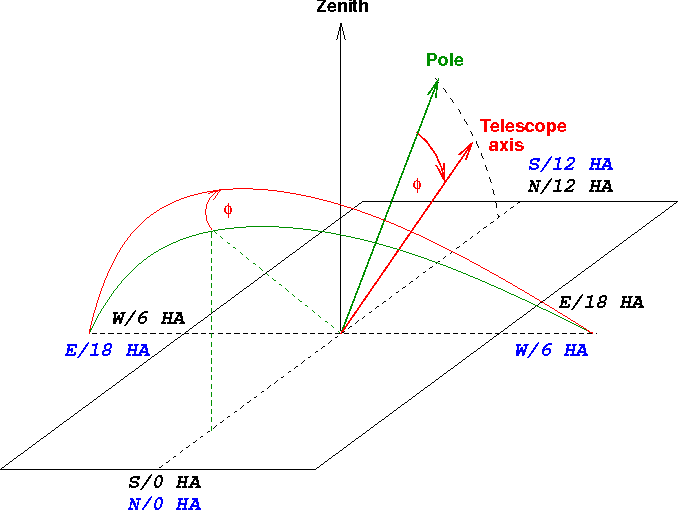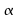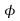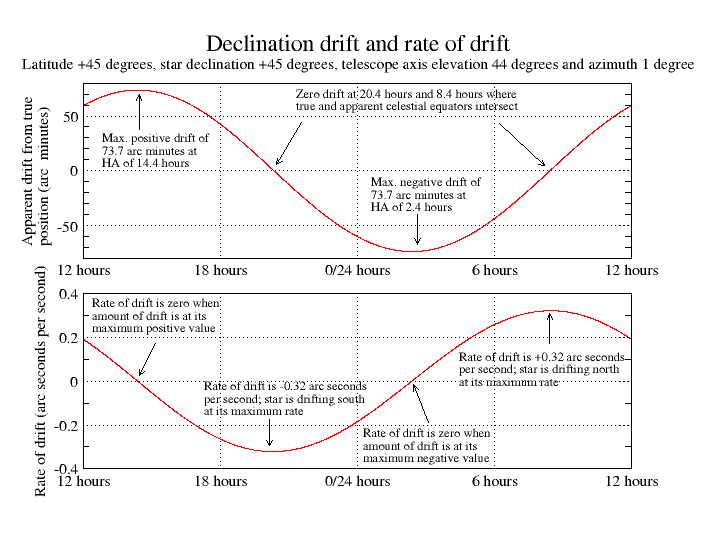# Canburytech.net

## Declination drift due to polar misalignment (no refraction)

Declination drift occurs when the polar axis of the telescope mount is not aligned with the true pole. A star follows a particular path across the sky as the earth rotates, but if the star is being tracked by a telesope whose mount is not aligned with the earth's rotational axis, then the star appears to drift in the north/south direction in the telescope's field of view. We can take advantage of this to align the telescope's polar axis. All we need to do is monitor the drift of the star and adjust the azimuth and elevation of the mount's polar axis until the drift is very small. (Polar misalignment also causes an east/west drift, but that's harder to measure reliably so we won't discuss it here). Now let's see how declination drift works in practice.

### Telescope axis offset in elevation

The following diagram shows the situation for the telescope's polar axis offset to below the pole, at an hour angle (HA) of 12 hours. The green semicircle represents the celestial equator - at right-angles to the direction of the pole - and the red semicircle represents the apparent celestial equator, at right-angles to the telescope's polar axis. The horizontal plane represents the earth's surface at the location of the telescope. Points of the compass are shown in black for the northern hemisphere and blue for the southern hemisphere. The hour angle is the number of hours since a star was last on the meridian and starts at 0 hours, progressing westward along the celestial equator, and finally approaching 24 hours as the star reaches the meridian again. In the diagram, the values of HA are positioned around the edges of the horizontal plane for convenience.Suppose the telescope is pointed at a star on the celestial equator at the eastern horizon, at an hour angle of 18 hours, where the true and apparent celestial equators intersect. As the star rises, it follows the green line. The telescope would naturally follow the red line, so the star appears to dip below the path that the telescope follows. In the northern hemisphere, this means that the star drifts south, whilst in the southern hemisphere it drifts north. On the meridian (indicated by the vertical dashed green line), the star appears to have drifted by its greatest amount, but the rate of drift is zero because the red and green lines are parallel at that point. On the horizon, the divergence between the red and green lines is at its steepest and the rate of drift is greatest there. Notice that the star appears to have drifted by its greatest amount at an hour angle equal to the hour angle of the telescope axis plus or minus 12 hours, and that the greatest drift rate is at an hour angle equal to the hour angle of the telescope axis plus or minus 6 hours.

To correct the elevation of the telescope mount's axis by drift alignment, we can monitor the drift of a star at an hour angle of 18 hours (or 6 hours) and adjust the elevation of the telescope axis (up or down, according to the direction of drift) until the rate of drift is zero. Then we would hope to have our telescope's polar axis at the same elevation as the true pole. (Actually this is likely to be true only in the absence of any atmosphere - more on that later).

### Telescope axis offset in azimuth

Here's the diagram for the telescope axis offset in azimuth only. In this case, the telescope axis is at the same elevation as the true pole (equal to the observer's latitude), but we've rotated it a small amount about the zenith by altering the azimuth adjustment of the mount. Once again, the green semicircle represents the true celestial equator and the red semicircle represents the apparent celestial equator:Small offsets in azimuth are equivalent to tipping the telescope axis over from the pole in approximately the 6 hour/18 hour direction. The inset diagram attempts to show why this is so. If we look down from the zenith (Z) we see that the telescope axis would be at the pole (P) when perfectly aligned. But an adjustment in azimuth moves it to point T in our example, which is at an hour angle of a bit more than 18 hours for the northern hemisphere (or slightly less than 6 hours for the southern hemisphere). Remember that the hour angle is measured along the celestial equator with the pole at its centre, while the azimuth is measured along the horizontal plane with the zenith at its centre.

Suppose we point the telescope at a star on the celestial equator near the meridian, near the 0 hours postion, and follow it westwards. The telescope follows the red line and the star follows the green line. In the above diagram for the northern hemisphere, the telescope axis is offset east of north and the star appears to drift south as it moves westwards. In the above diagram for the southern hemisphere, the telescope axis is offset west of south and the star also appears to drift south as it moves westwards. When the star is almost due west, it appears to have drifted by the greatest amount from its true position. (This is also true when the star is almost due east; in this case the drift is in the opposite sense). The red and green lines diverge most steeply near the meridian close to an hour angle of zero, so the rate of drift is greatest there.

Remember that in this example the telescope's polar axis is pointing very close to the 18 hour direction for the northern hemisphere, or the 6 hour direction for the southern hemisphere. So once again we see that the star appears to have drifted by its greatest amount at an hour angle equal to the hour angle of the telescope axis plus or minus 12 hours (i.e. when the star is at 6 hours or 18 hours), but the greatest drift rate is at an hour angle equal to the hour angle of the telescope axis plus or minus 6 hours (i.e. when the star is at 0 hours or 12 hours).

To correct the azimuth of the telescope axis by drift alignment, we can monitor the drift of a star on the meridian and adjust the azimuth of the telescope axis (east or west, according to the direction of drift) until the rate of drift is zero. Then we would expect the telescope's polar axis to lie due north/south.

There's a subtlety about the azimuth adjustment that we should note. When we adjust the azimuth of the telescope mount, we are altering the angle. But the offset of the telescope mount's axis from the pole is the angle, and these two angles are the same only at the equator; generally,is less than. (In fact we have to multiplyby the cosine of our latitude to get). So although an offset of 1 degree in elevation really does produce a 1 degree difference between the pole and the telescope axis, an offset of 1 degree in azimuth generally does not.

This table shows how the offset of the telescope mount's axis from the pole varies with latitude, for a fixed azimuth error of 1 degree:

Latitude (degrees)Polar offset (arc min)
060.00
+/-3051.96
+/-4542.43
+/-6030.00

In other words, 1 degree of azimuth offset at 45 degrees latitude is no worse than 43 arc minutes offset in elevation. This is worth bearing in mind of you have a telescope controller that gives you the offset from the pole as an azimuth and elevation error, or if you are comparing the apparent accuracy of your polar alignment with someone at a different latitude.

### Telescope axis offset in elevation and azimuth

In general, the telescope axis will be offset in azimuth and elevation, so the true situation will be a combination of the above two diagrams. We can study these situations with the help of the spreadsheet. Here, as an example, is the declination drift and the rate of drift for a latitude of +45 degrees with the telescope mount's polar axis set to an elevation of 44 degrees and an azimuth of 1 degree. The curves are drawn for a star with a declination of +45 degrees (so it's just circumpolar, reaching the zenith on the meridian and the horizon when due north).The spreadsheet tells us that the size of the offset from the pole is 73.7 arc minutes at an hour angle of 14.4 hours. Exactly as we noted from the above diagrams, the maximum drift (73.7 arc minutes) occurs at the same hour angle as the direction of the telescope axis relative to the pole (i.e. at an hour angle of 14.4 hours) and also directly opposite in the sky (at 2.4 hours). The greatest drift rate occurs at plus or minus 6 hours from the direction of inclination of the axis (i.e. at 8.4 and 20.4 hours). The maximum rate of drift is 0.32 arc seconds per second and using the formula for maximum drift rate given on the equations page, we can deduce that the maximum offset from the pole must be (0.32 x 24 x 3600) / () arc seconds, i.e. 4400 arc seconds, or about 73.4 arc minutes, in excellent agreement with the exact spreadsheet calculation.

Usually we would hope to have the telescope axis aligned closer to the pole than this to give us good tracking accuracy over long periods. But note what happens as we shrink the distance of the polar offset: at an offset of just five arc minutes, the maximum declination drift rate shrinks to about 0.02 arc seconds per second, or one arc second every 50 seconds of time. So we need to observe for long enough to be able to detect that sort of drift reliably and we must make absolutely certain that any other sources of apparent drift (such as mirror flop or flexure in the imaging train) are much smaller than this.

### Direction of drift

Drift alignment still works even when the telescope axis is misaligned in both elevation and azimuth. Although the maximum drift rate won't be exactly at the meridian or 6 hour/18 hour locations, we can still reduce the drift at the meridian until it is as small as we like by adjusting the azimuth. Even if the elevation is wrong, the drift rate at the meridian will still be zero (or very small) when the telescope mount's polar axis is aligned with the meridian. We can then reduce the drift at 6/18 hours by adjusting the elevation.

With the help of the above diagrams, it is straightforward to work out the direction of drift for a given offset and the corrections we need to make. These are summarised in the following tables:

HemisphereStar on meridian (0 hours) drifts this way:Polar axis points this way:Adjust polar axis this way:
NorthernNorthWest of due NorthMove axis East
NorthernSouthEast of due NorthMove axis West
SouthernNorthEast of due SouthMove axis West
SouthernSouthWest of due SouthMove axis East

HemisphereStar easterly (18 hours) drifts this way:Polar axis points this way:Adjust polar axis this way:
NorthernNorthAbove the poleLower the axis
NorthernSouthBelow the poleRaise the axis
SouthernNorthBelow the poleRaise the axis
SouthernSouthAbove the poleLower the axis

HemisphereStar westerly (6 hours) drifts this way:Polar axis points this way:Adjust polar axis this way:
NorthernNorthBelow the poleRaise the axis
NorthernSouthAbove the poleLower the axis
SouthernNorthAbove the poleLower the axis
SouthernSouthBelow the poleRaise the axis

Unfortunately, we have so far been ignoring a problem that becomes apparent if we attempt to adjust the orientation of the telescope axis to within a few arc minutes of the pole. The source of our difficulty is refraction caused by the earth's atmosphere. We can still make adjustments as described in the tables above, but we find that in practice we have not aligned the telescope axis with the true pole. Let's have a look at that next.